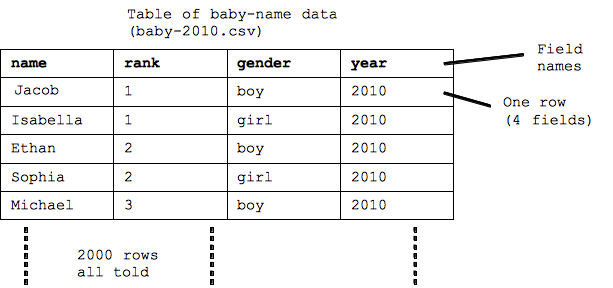# Table-1 Data

• table - next data format for CS101
• Re-use the code idioms, loops etc. from images
• Tables are a very common way to organize data on the computer

As another example of how data is stored and manipulated in the computer, we'll look at "table data" -- a common a way to organize strings, numbers, dates in rectangular table structure. In particular, we'll start with data from the social security administration baby name site.

## Social Security Baby Name Table

• Names for babies born each year in the USA
• Top 1000 boy and girl names, 2000 names total
• Table terminology:
- table the whole rectangle of data
- row data for one name
- field individual items (columns) in a row,
• Each field has a name: name, rank, gender, year## Tables Are Extremely Common

• The rectangular table data format is very common
• "Database" - extension of this basic table idea
• Number of fields is small (categories)
• Number of rows can be millions or billions
-e.g. email inbox: one row = one message, fields: date, subject, from, ...
-e.g. craigslist: one row = one thing for sale: description, price, seller, date, ...
• Demo craigslist search, list output mode
-Craigslist has a 100 million "rows" , queries out 20 to show us

Much of the information stored on computers uses this table structure. One "thing" we want to store -- a baby name, someone's contact info, a craigslist advertisement -- is one row. The number of fields that make up a row is fairly small -- essentially the fixed categories of information we think up for that sort of thing. For example one craigslist advertisement (stored in one row) has a few fields: a short description, a long description, a price, a seller, ... plus a few more fields.

The number of fields is small, but the number of rows can be quite large -- thousands or millions. When someone talks about a "database" on the computer, that builds on this basic idea of a table. Also storing data in a spreadsheet typically uses exactly this table structure.

## Table Code

We'll start with some CS101 code -- SimpleTable -- which will serve as a foundation for you to write table code. Run the code to see what it does.

• Baby data stored in "baby-2010.csv"
• ".csv" stands for "comma separated values"
- csv is a simple format to store a table as a text file
• Recall we had: `for (pixel: image) { code`
• For tables: `for (row: table) { code`
• print(row) prints all the fields of a row on one line
• (Reload the page to get rid of the printing, or // comment out print)

table-1-1

## Extract Field From Row - getField()

• Field names for the baby table: name, rank, gender, year
• `row.getField("field-name")` -- pick field out of row
• Q: What do you think the code below will print?

table-1-2

## Table Query 1 - Rank

• Use if-statement to select certain rows
• A "query" in database terminology
• e.g. select rows where the rank is 6
`if (row.getField("rank") == 6) { ...`
• Equality test `==` (2 equal signs)
• Loop runs for every rows (2000), if picks out some
• Aside: there are other ways to pick data out of many rows, this is the way we will use
e.g. if there were 100 million rows, loop is not the best

table-1-3

## Table Query 2 - Name

The above code loops over all the rows, and the if-statement prints just the rows where the test is true, here testing if the rank field is equal to 6, but really the if-statement could test anything about the row.

• Field names for the baby table: name, rank, gender, year
• `row.getField("field-name")`
• (demo) e.g. select row where the name is "Alice"
`if (row.getField("name") == "Alice") { ...`
• (demo) Warning: single equal sign `=` does variable assignment, not comparison. Use `==` inside if-test.
• Other comparisons: < > <= >= (below)

table-1-4

The row object has a `row.getField("field-name")` function which returns the data for one field out of the row. Each field has a name -- one of "name" "rank" "gender" "year" in this case -- and the string name of the field is passed in to getField() to indicate which field we want, e.g. `row.getField("rank")` to retrieve the rank out of that row.

You can test if two values are equal in JavaScript with two equal signs joined like this: `==`. Using ==, the code to test if the name field is "Alice" is `row.getField("name") == "Alice"`

Note that a single equal sign `=` does variable assignment and not comparison. It's a common mistake to type in one equal sign for a test, when you mean two equal signs. For this class, the Run button will detect an accidental use of a single = in an if-test and give an error message. The regular less-than/greater-than type tests: < > <= >= work as have seen before.

## Table Query Examples

• Equality test "operator" `==`
• Other comparison operators:
< (less than)
> (greater than)
<= (less than or equal)
>= (greater than or equal)

table-1-5

Example queries, and some you-try-it

• Baby table fields: name, rank, gender, year
• name field is "Robert", "Bob", "Abby", "Abigail" (try each in turn, yes nobody names their child "Bob" .. apparently always using Robert or Bobby)
• rank field is 1
• rank field is < 10
• rank field is <= 10
• rank field is > 990
• gender field is "girl"
• You try it:
• rank field is less than 15
• gender field is "boy"
• In all cases:
The loop goes through all 2000 rows and evaluates the if-test for each, printing that row only if the test is true.

Solution code: